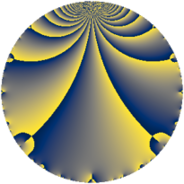Properties

 Label 4560.2.a.beLevel $4560$ Weight $2$ Character orbit 4560.a Self dual yes Analytic conductor $36.412$ Analytic rank $1$ Dimension $2$ CM no Inner twists $1$

Related objects

Newspace parameters

 Level: $$N$$ $$=$$ $$4560 = 2^{4} \cdot 3 \cdot 5 \cdot 19$$ Weight: $$k$$ $$=$$ $$2$$ Character orbit: $$[\chi]$$ $$=$$ 4560.a (trivial)

Newform invariants

 Self dual: yes Analytic conductor: $$36.4117833217$$ Analytic rank: $$1$$ Dimension: $$2$$ Coefficient field: $$\Q(\sqrt{5})$$ Defining polynomial: $$x^{2} - x - 1$$ Coefficient ring: $$\Z[a_1, \ldots, a_{7}]$$ Coefficient ring index: $$2$$ Twist minimal: no (minimal twist has level 2280) Fricke sign: $$1$$ Sato-Tate group: $\mathrm{SU}(2)$

$q$-expansion

Coefficients of the $$q$$-expansion are expressed in terms of $$\beta = \sqrt{5}$$. We also show the integral $$q$$-expansion of the trace form.

 $$f(q)$$ $$=$$ $$q - q^{3} - q^{5} + ( -3 - \beta ) q^{7} + q^{9} +O(q^{10})$$ $$q - q^{3} - q^{5} + ( -3 - \beta ) q^{7} + q^{9} + ( -1 + \beta ) q^{11} + ( 3 - \beta ) q^{13} + q^{15} + 2 \beta q^{17} - q^{19} + ( 3 + \beta ) q^{21} + ( 2 - 2 \beta ) q^{23} + q^{25} - q^{27} + ( 3 - \beta ) q^{29} + 4 \beta q^{31} + ( 1 - \beta ) q^{33} + ( 3 + \beta ) q^{35} + ( 1 + \beta ) q^{37} + ( -3 + \beta ) q^{39} + ( -3 - 3 \beta ) q^{41} + ( -3 - \beta ) q^{43} - q^{45} + ( 2 - 2 \beta ) q^{47} + ( 7 + 6 \beta ) q^{49} -2 \beta q^{51} + ( 4 - 2 \beta ) q^{53} + ( 1 - \beta ) q^{55} + q^{57} + ( 6 + 2 \beta ) q^{59} + 2 \beta q^{61} + ( -3 - \beta ) q^{63} + ( -3 + \beta ) q^{65} + ( -6 + 2 \beta ) q^{67} + ( -2 + 2 \beta ) q^{69} + ( 8 + 2 \beta ) q^{73} - q^{75} + ( -2 - 2 \beta ) q^{77} + q^{81} -4 q^{83} -2 \beta q^{85} + ( -3 + \beta ) q^{87} + ( 5 - 3 \beta ) q^{89} -4 q^{91} -4 \beta q^{93} + q^{95} + ( -7 + \beta ) q^{97} + ( -1 + \beta ) q^{99} +O(q^{100})$$ $$\operatorname{Tr}(f)(q)$$ $$=$$ $$2 q - 2 q^{3} - 2 q^{5} - 6 q^{7} + 2 q^{9} + O(q^{10})$$ $$2 q - 2 q^{3} - 2 q^{5} - 6 q^{7} + 2 q^{9} - 2 q^{11} + 6 q^{13} + 2 q^{15} - 2 q^{19} + 6 q^{21} + 4 q^{23} + 2 q^{25} - 2 q^{27} + 6 q^{29} + 2 q^{33} + 6 q^{35} + 2 q^{37} - 6 q^{39} - 6 q^{41} - 6 q^{43} - 2 q^{45} + 4 q^{47} + 14 q^{49} + 8 q^{53} + 2 q^{55} + 2 q^{57} + 12 q^{59} - 6 q^{63} - 6 q^{65} - 12 q^{67} - 4 q^{69} + 16 q^{73} - 2 q^{75} - 4 q^{77} + 2 q^{81} - 8 q^{83} - 6 q^{87} + 10 q^{89} - 8 q^{91} + 2 q^{95} - 14 q^{97} - 2 q^{99} + O(q^{100})$$

Embeddings

For each embedding $$\iota_m$$ of the coefficient field, the values $$\iota_m(a_n)$$ are shown below.

For more information on an embedded modular form you can click on its label.

Label $$\iota_m(\nu)$$ $$a_{2}$$ $$a_{3}$$ $$a_{4}$$ $$a_{5}$$ $$a_{6}$$ $$a_{7}$$ $$a_{8}$$ $$a_{9}$$ $$a_{10}$$
1.1
 1.61803 −0.618034
0 −1.00000 0 −1.00000 0 −5.23607 0 1.00000 0
1.2 0 −1.00000 0 −1.00000 0 −0.763932 0 1.00000 0
 $$n$$: e.g. 2-40 or 990-1000 Significant digits: Format: Complex embeddings Normalized embeddings Satake parameters Satake angles

Atkin-Lehner signs

$$p$$ Sign
$$2$$ $$1$$
$$3$$ $$1$$
$$5$$ $$1$$
$$19$$ $$1$$

Inner twists

This newform does not admit any (nontrivial) inner twists.

Twists

By twisting character orbit
Char Parity Ord Mult Type Twist Min Dim
1.a even 1 1 trivial 4560.2.a.be 2
4.b odd 2 1 2280.2.a.p 2
12.b even 2 1 6840.2.a.bd 2

By twisted newform orbit
Twist Min Dim Char Parity Ord Mult Type
2280.2.a.p 2 4.b odd 2 1
4560.2.a.be 2 1.a even 1 1 trivial
6840.2.a.bd 2 12.b even 2 1

Hecke kernels

This newform subspace can be constructed as the intersection of the kernels of the following linear operators acting on $$S_{2}^{\mathrm{new}}(\Gamma_0(4560))$$:

 $$T_{7}^{2} + 6 T_{7} + 4$$ $$T_{11}^{2} + 2 T_{11} - 4$$ $$T_{13}^{2} - 6 T_{13} + 4$$ $$T_{17}^{2} - 20$$

Hecke characteristic polynomials

$p$ $F_p(T)$
$2$ $$T^{2}$$
$3$ $$( 1 + T )^{2}$$
$5$ $$( 1 + T )^{2}$$
$7$ $$4 + 6 T + T^{2}$$
$11$ $$-4 + 2 T + T^{2}$$
$13$ $$4 - 6 T + T^{2}$$
$17$ $$-20 + T^{2}$$
$19$ $$( 1 + T )^{2}$$
$23$ $$-16 - 4 T + T^{2}$$
$29$ $$4 - 6 T + T^{2}$$
$31$ $$-80 + T^{2}$$
$37$ $$-4 - 2 T + T^{2}$$
$41$ $$-36 + 6 T + T^{2}$$
$43$ $$4 + 6 T + T^{2}$$
$47$ $$-16 - 4 T + T^{2}$$
$53$ $$-4 - 8 T + T^{2}$$
$59$ $$16 - 12 T + T^{2}$$
$61$ $$-20 + T^{2}$$
$67$ $$16 + 12 T + T^{2}$$
$71$ $$T^{2}$$
$73$ $$44 - 16 T + T^{2}$$
$79$ $$T^{2}$$
$83$ $$( 4 + T )^{2}$$
$89$ $$-20 - 10 T + T^{2}$$
$97$ $$44 + 14 T + T^{2}$$